# Corresponding Angles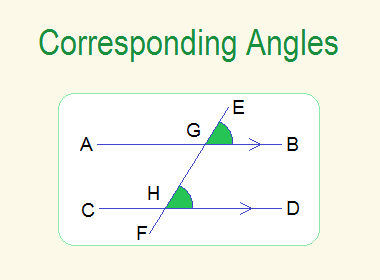## Corresponding Angles

When two lines are cut by a transversal, then a pair of non-adjacent angles on the same side of a transversal, one external and the other internal are called corresponding angles.

**********************

10 Math Problems officially announces the release of Quick Math Solver, an android APP on the Google Play Store for students around the world.**********************

In the figure given below, transversal EF has been cut to the two non-parallel lines AB and CD at G and H.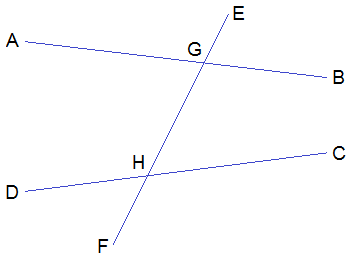Here, the corresponding angles are:

i.             AGE and GHD

ii.          AGH and DHF

iii.       EGB and GHC

iv.        BGH and CHFIf two parallel lines are cut by a transversal then the corresponding angles are equal. In below figure, transversal RS has been cut to the two parallel lines MN and PQ at A and B.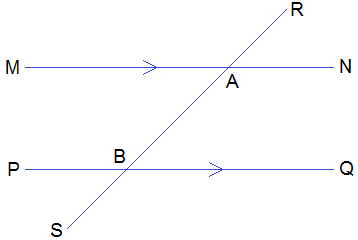So, the corresponding angles are equal. i.e.

i.             RAM = ABP

ii.          MAB = PBS

iii.       RAN = ABQ

iv.        NAB = QBS

Worked Out Examples

Example 1: Find the value of x from the given figure.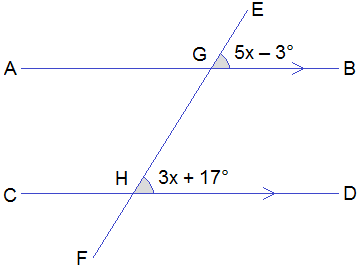Solution:

Here,

From the figure,

5x – 3° = 3x + 17° ------> Corresponding angles.

or,     5x – 3x = 17° + 3°

or,     2x = 20°

or,     x = 20°/2

or,     x = 10° Ans.

Example 2: Find the value of a and b from the given figure.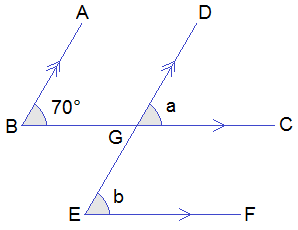Solution:

Here,

From the figure,

a = 70° ------> Corresponding angles.

b = a ------> Corresponding angles.

= 70°

a = 70° and b = 70° Ans.

Example 3: Find the value of a, b and c from the given figure.Solution:

Here,

From the figure,

a = 50° ------> Corresponding angles.

b = 70° ------> Corresponding angles.

Now,

c + 50° + 70° = 180° ------> Sum of angles of a Δ.

or,     c + 120° = 180°

or,     c = 180° – 120°

or,     c = 60°

a = 50°, b = 70° and c = 60° Ans.

If you have any questions or problems regarding the Corresponding Angles, you can ask here, in the comment section below.